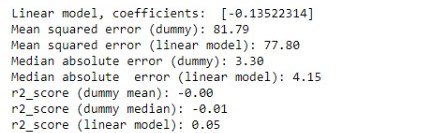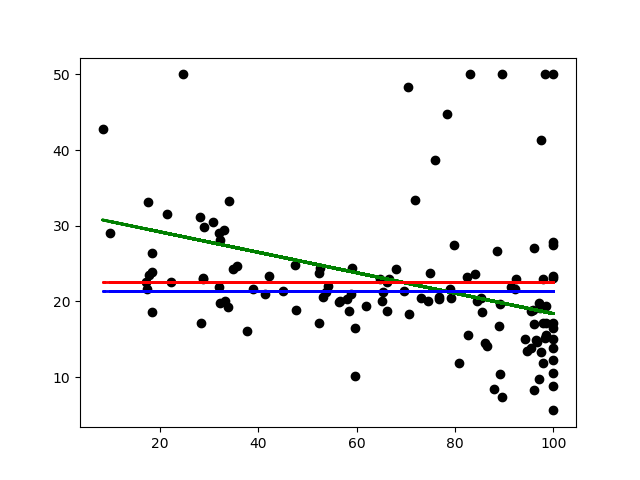GeeksforGeeks App
Open AppBrowser
Continue

## Related Articles

The Dummy Regressor is a kind of Regressor that gives prediction based on simple strategies without paying any attention to the input Data. As similar to Dummy Classifier the sklearn library also provides Dummy Regressor which is used to set up a baseline for comparing other existing Regressor namely  Poisson Regressor, Linear Regression, Ridge Regression and many more. However, in this article, the main focus will be to draw a comparison between Dummy Regression and Linear regression.

• STEP 1- Importing the necessary modules. The dummy module of sklearn provides an in-built DummyRegressor model which will be used in this case. Apart from importing other modules the mean square error and the median absolute error are worth special mentioning and the purpose of doing so will be explained later in the due course.

## Python3

 `import` `matplotlib.pyplot as plt``import` `numpy as np``from` `sklearn.model_selection ``import` `train_test_split``from` `sklearn ``import` `datasets``from` `sklearn.linear_model ``import` `LinearRegression``from` `sklearn.metrics ``import` `mean_squared_error, r2_score, median_absolute_error``from` `sklearn.dummy ``import` `DummyRegressor`

• STEP 2- Loading the Dataset. Here the Boston Dataset has been used for the purpose which is available in the sklearn                   Dataset module. Since, this is a regression problem, so only one feature i.e. the “data” has been considered as an input feature and labelled as X and “target” as y for the target labels. To match the X and y dimensionality the X is reduced to 1 element in each row, by the following code.

## Python3

 `boston``=``datasets.load_boston()``X``=``boston.data[:, ``None``, ``6``]``y``=` `boston.target`

• STEP 3-  Training and testing the dummy and the linear model.  The below code shows that training the dummy model is similar to training any regular regression model, except for the strategies. The main role of strategy is to predict target values without any influence of the training data. There are namely four types of strategies that are used by the Dummy Regressor:-
1. Mean: This is the default strategy used by the Dummy Regressor. It always predicts the mean of the training target values.
2. Median: This is used to predict the median of the training target values.
3. Quantile: It is used to predict a particular quantile of training target values provided the quantile parameter is used along with it.
4. Constant: This is generally used to predict a specific custom value that is provided and the constant parameter must be mentioned.

## Python3

 `X_train, X_test, y_train, y_test ``=` `train_test_split(X, y, random_state``=``0``)``lm ``=` `LinearRegression().fit(X_train, y_train)``lm_dummy_mean ``=` `DummyRegressor(strategy ``=` `'mean'``).fit(X_train, y_train)`` ` `lm_dummy_median ``=` `DummyRegressor(strategy ``=` `'median'``).fit(X_train, y_train)``y_predict ``=` `lm.predict(X_test)``y_predict_dummy_mean ``=` `lm_dummy_mean.predict(X_test)``y_predict_dummy_median ``=` `lm_dummy_median.predict(X_test)`

In this case, however, the “mean” and “median” has been used for strategy. But the other two can be used depending upon the necessity.

After training both the models they are evaluated on the test set using y_predict   for the linear model and y_predict_dummy_mean and y_predict_dummy_median for predicting the dummy mean and median respectively.

• STEP 4- Error analysis.  To understand how better both the model performed the evaluation metrics such as mean squared error, median absolute error and r2_ score are calculated for both the linear and dummy models. The mean square error and the median absolute error are evaluated along with r2_ score, mainly to demonstrate the influence of “mean”, “median” strategies of the DummyRegressor.

## Python3

 `print``(``'Linear model, coefficients: '``, lm.coef_)``print``(``"Mean squared error (dummy): {:.2f}"``.``format``(mean_squared_error(y_test, ``                                                                     ``y_predict_dummy_mean)))``print``(``"Mean squared error (linear model): {:.2f}"``.``format``(mean_squared_error(y_test, y_predict)))`` ` `print``(``"Median absolute error (dummy): {:.2f}"``.``format``(median_absolute_error(y_test, ``                                                                    ``y_predict_dummy_median)))``print``(``"Median absolute error (linear model): {:.2f}"``.``format``(median_absolute_error(y_test, y_predict)))`` ` `print``(``"r2_score (dummy mean): {:.2f}"``.``format``(r2_score(y_test, y_predict_dummy_mean)))``print``(``"r2_score (dummy median): {:.2f}"``.``format``(r2_score(y_test, y_predict_dummy_median)))``print``(``"r2_score (linear model): {:.2f}"``.``format``(r2_score(y_test, y_predict)))`

Output-Error Analysis

OBSERVATION: As can be seen from the above result. The expected Dummy Regressor always predict the r2_ score as 0 for both the mean and median, since it is always predicting a constant without having an insight of the output. ( In general, best r2_score is 1 and Constant r2_score is 0). The Linear Regression Model seems to fit a little better than the Dummy Regressor in terms of “mean squared error”, “median absolute error” and “r2_score”.

• STEP 5- For visualizing the performance of Dummy Regressor and Linear Regressor, both the models are plotted over the test data.

## Python3

 `plt.scatter(X_test, y_test,  color``=``'black'``)``plt.plot(X_test, y_predict, color``=``'green'``, linewidth``=``2``)``plt.plot(X_test, y_predict_dummy_median, color``=``'blue'``, linestyle ``=` `'dashed'``, ``         ``linewidth``=``2``, label ``=` `'dummy'``)``plt.plot(X_test, y_predict_dummy_mean, color``=``'red'``, linestyle ``=` `'dashed'``, ``         ``linewidth``=``2``, label ``=` `'dummy'``)`Plot of  data vs target

Conclusion- The scattered plot in the above plot, are the instances of the test set that trends to accumulate slightly at the bottom right. The green line is the linear regression model that was fit to the training points. The red line shows the dummy mean that always uses the strategy of predicting the mean of the training set, similarly the blue line shows the dummy median and serves the same purpose for predicting the median of the training set. As it is, see the linear model doesn’t fit so well to test data.

Hence, it can now be finally concluded that the Dummy Regressor can be used for checking how well a regular regression model is fitted to a particular dataset but can never be used in any real problem.

Please refer to the scikit learn documentation(“https://scikit-learn.org/stable/modules/generated/sklearn.dummy.DummyRegressor.html“) for more details.

My Personal Notes arrow_drop_up